# 暗黑3魔法师技能测试：独立增伤的原力之波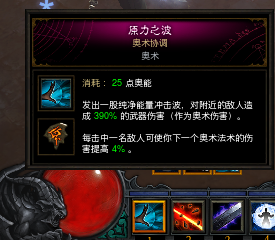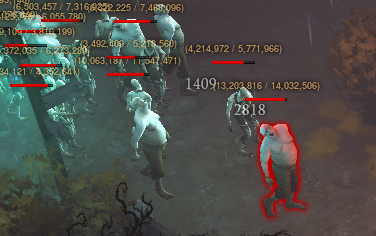2818/1648=1.709≈1.72=1+（0.04*18）满足增伤系数。

1949/1648=1.182≈1.2

3394/1949=1.74≈1.72=1+（0.04*18）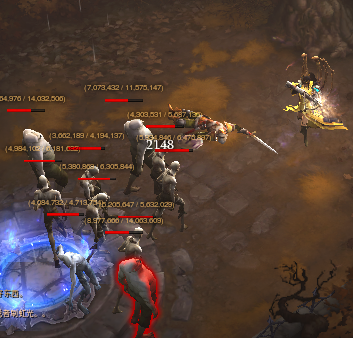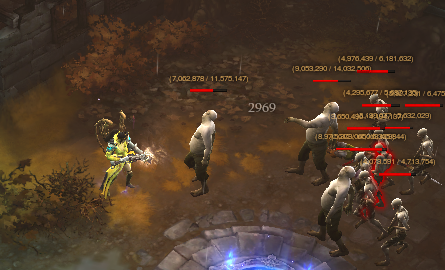2969/2148=1.3822≈1.4=1+(0.04*10)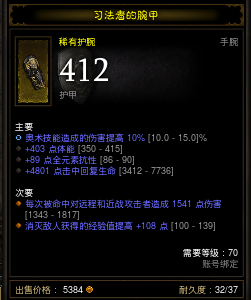3486/2163=1.61≈1.60=1+0.04*15

### 跟帖0条跟帖

#### 最新跟帖

跟帖成功，查看我的跟帖

### 意见反馈

网易公司版权所有